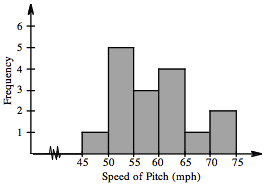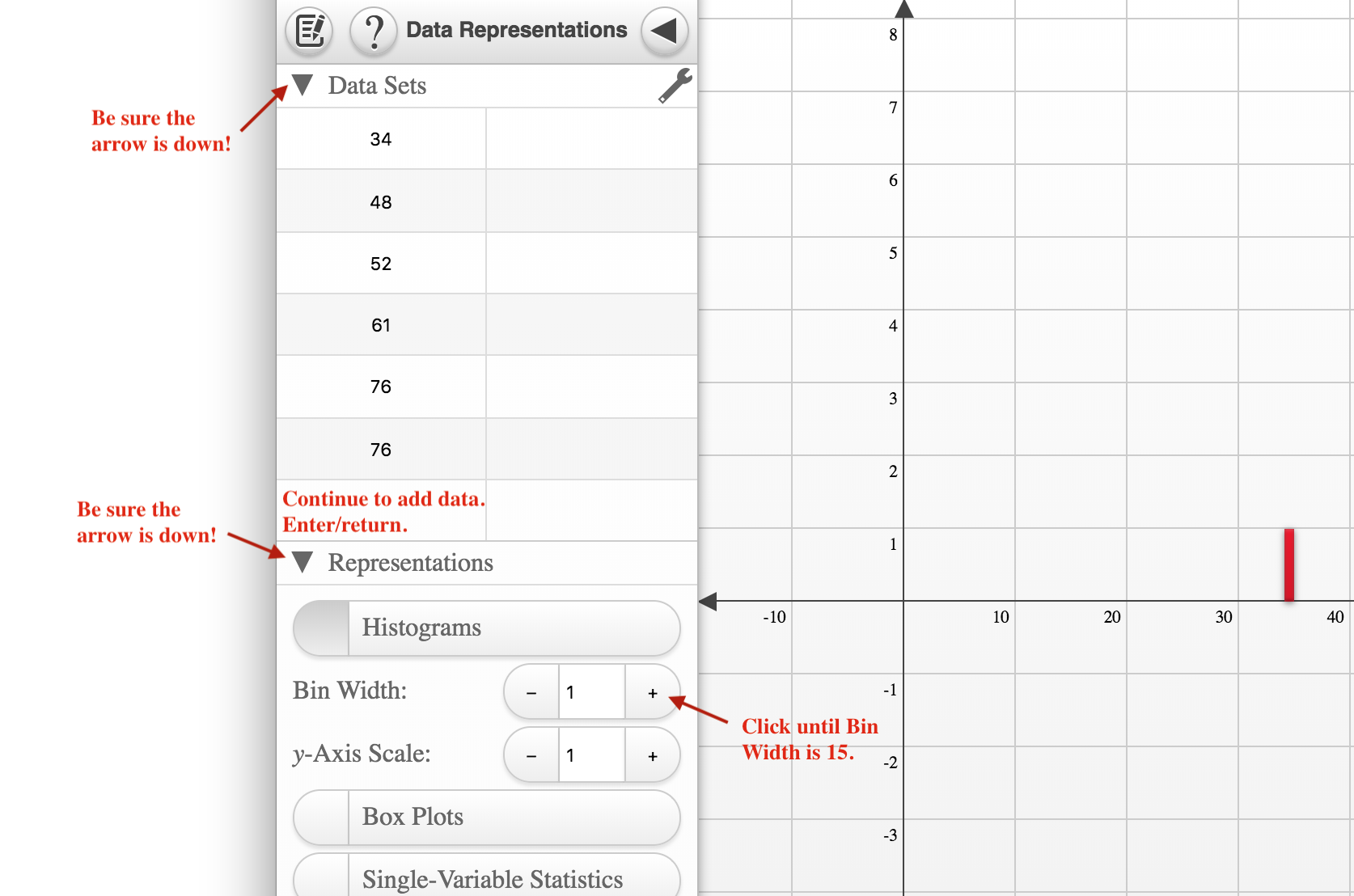### Home > CC2 > Chapter 2 > Lesson 2.2.4 > Problem2-84

2-84.

Jahna measured the heights of the sunflowers growing in her backyard. Here are the heights that she found (in inches): $34$, $48$, $52$, $61$, $76$, $76$, $61$, $84$, $61$, $39$, $83$, $61$, $79$, $81$, $56$, and $88$. Find the mean and median of the heights. 2-84 HW eTool (CPM). Homework Help ✎

1. Find the mean and median of the heights.

The mean is the sum of all the values divided by the number of values.

If all the values are arranged from least to greatest, the median is the value occupying the middle position.
If there are an even number of values, the median is the mean of the two middle values.

$\text{Mean}=65$, $\text{Median}=61$

2. Create a histogram to represent this data. Your histogram should have four bins, each with a width of $15$.

In this histogram, there are $6$ bins and they each have a width of $5$.

Do you remember how to make a histogram? See the example at right from problem 1-65.Enter the data in the eTool below to find the mean and median and create a histogram.
Click the link at right for the full version of the eTool: CC2 2-84 HW eTool